Start Now with 100 Free Questions

# CAT4 – Nonverbal Reasoning

## What includes in the CAT4 Nonverbal Reasoning?

The CAT4 Nonverbal Reasoning section is composed of subtests that assess a student’s ability to think logically and analytically without relying on language skills.

The specific subtests included in the Nonverbal Reasoning section may vary depending on the level of the test and the testing organization, but generally, figure classification and figure matrices are included. These subtests, along with examples, are explained below.

## Figure Classification

This subtest presents students with three figures. The student must identify the conceptual link or underlying characteristic that the three figures have in common.  Then they must select a figure from the answers that share the same characteristics and belongs with the first three figures.

Instruction:  The first 3 figures on the left relate to each other in some way.  Find a figure on the right of the line that goes with the first 3 figures in exactly the same way.

Example 1: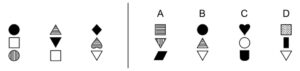Answer:  B – Each figure has one solid black, one solid white, and one striped shape.

Example 2: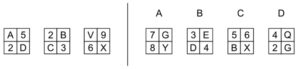Answer:  B – The letters and numbers are diagonally across from each other.

Instruction:  Look at the 3 figures on top. They are related in some way. Choose an answer on the bottom that goes with the first 3 figures in exactly the same way.

Example 3: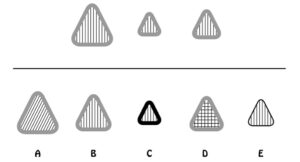Answer:  B – The figure has the same outer border and same inside pattern as the 3 figures on top.

Example 4: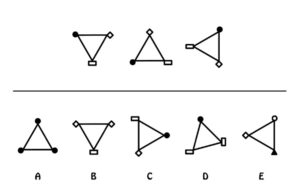Answer:  C – All of the figures have the same small shape (a black circle, a white quadrangle, and a white diamond).

## Figure Matrices

This subtest presents the student with two figures that are related in some way. Students must work out the relationship between the first two figures, then select an answer that belongs with the third figure the same way the first two figures belong together.

Examples of Figure Matrices questions are below from lower-level questions to higher-level questions.

Instruction:  Look at the figures on top. They go together in some way. Choose a figure from the answer row that goes with the figure(s) on the bottom the same way the figures on top go together.

Example 1: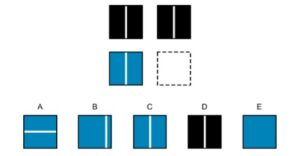Answer:  C – The top two figures are the same. Therefore, answer C makes the bottom two figures the same.

Example 2: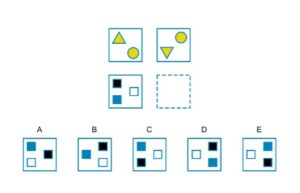Answer:  C – The shapes on bottom go up and turn upside down, shapes on top go down and turn upside down; shape in middle stays put – it doesn’t matter that shapes turn upside down with the second row because the shapes are square.

Example 3: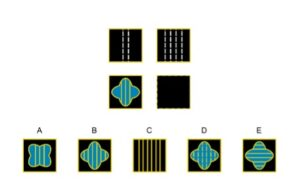Answer:  B – The number of lines in the design doubles.

Example 4: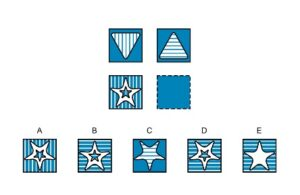Answer:  D –The stripes go from vertical to horizontal; the shape turns upside down.

Overall, the Nonverbal Reasoning section of the CAT4 is designed to assess a student’s ability to think logically and analytically without relying on language skills. The questions in this section may involve recognizing patterns and relationships between figures, analyzing and manipulating visual stimuli to identify the pattern or rule that governs a series of figures, and identifying the missing figure that completes an analogy.